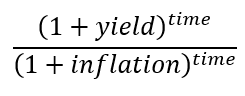## Thursday, December 7, 2017

Published December 07, 2017 by with 0 comment

# How Long Does It Take To Double Your Money With An Investment If You Include Inflation?

In a previous post, I worked through why dividing 72 by your interest rate approximates your doubling time in years.
Inflation makes everything more expensive. It lowers the purchasing power of each dollar you have, so just because an investment doubles in number of dollars, it doesn't necessarily double in value. How do you calculate this effect?

An incorrect way that might seem obvious is just to subtract inflation rate from your interest rate. E.g., if you gain 15%, and inflation is 5%, then subtract 5 from 15 to get a yield of 10%. That doesn't actually work.

Consider that simple example and look at the actual numbers:

The difference here is maybe easiest to understand by considering how much of an item you can buy. Say an item costs \$100 today, and you have \$100. You can buy exactly one of those items (\$100/\$100 = 1).

Now, say you invested that \$100. You got 15% on your investment somehow, and inflation was 5%. After 10 years, you have \$405 (1.15^10) and the item costs \$163 (1.05^10). You can now buy (\$405/\$163) = ~2.5 of the items. If you were to do this by subtracting the inflation rate from the yield, you'd have \$260 (1.1^10) so you could buy ~2.6 of the items.

Taking away the specific numbers, the effective power of the money from that example is:Equation 1: This is the correction equation

Simplified a bit if you prefer, that's:Equation 2: This is a simplified version of the correct equation

If you represent the 'yield - inflation' method above, you get this:Equation 3: This is the incorrect equation

Those two are not equivalent.

Now...to actually calculate the doubling time. The equation above gives you the effective yield. Referring to the previous post on this, we found:Equation 4 \

where t is the doubling time and r is the effective yield. Combining this with Equation 2 then gives you the actual doubling time:Equation 5

You can just plug in numbers that you want. I've put a few examples below:

 gain % inflation % doubling time (years) 5 0 14.2 5 2 23.9 8 0 9.0 8 2 12.1 10 0 7.3 10 2 9.2

Important note...remember that rates are often given in %. Thus, an 8% yield means the yield in the equations above is 0.08.